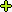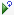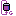# Tutorial: Create and Train a Sigmoid based Policy Gradient RL Model on an ATARI Pong Gym

This tutorial will guide you through the steps to create a Sigmoid based Policy Gradient Reinforcement Learning model as described by Andrej Karpathy  and train it on the ATARI gym with the ATARI game ‘Pong’ which uses the AleControl to access the Arcade-Learning-Environment  which is based on the ATARI 2600 Emulator created by The Stella Team .

##### Step 1 – Create the Project

In the first step we need to create a new project that contains the ATARI gym, the simple Policy Gradient model and the RMSProp solver.

To do this, first select the Add Project () button at the bottom of the Solutions window, which will display the New Project dialog.

To add the ATARI gym, press the Browse (…) button to the right of the Dataset: field which will display the Datasets dialog.

Next, add the ATARI model and solver using the Browse (…) button next to the Model: and Solver: fields.

Upon selecting OK on the New Project dialog, the new PongTest project will be displayed in the Solutions window.

##### Step 2 – Review the Model

Now that you have created the project, lets open up the model to see how it is organized. To review the model, double click on the SimpleNetAtari model within the new PongTest project, which will open up the Model Editor window.

As you can see, the policy gradient reinforcement learning model used is fairly simple, comprising of just two inner product layers.  NOTE: The data layer has a size of 10, 1, 80, 80 which specifies to use a batch size of 10 data items each with a single channel (black and white) with a height x width of 80 x 80.

A MemoryData layer feeds the batches of 10 items, received on each step of the ATARI simulation, into the model. The data values used on each step are a series of black and white dots where white is used to color the locations of the paddles and ball as the game is played.

The actual data used is a difference created between the current step and the previous step, which is then fed into the MemoryData layer and proceeds on down the model during the forward pass.

The Sigmoid layer provides the network output which is treated as a probability used to determine whether to move the paddle up or down.

The MemoryLoss layer calculates the loss and gradients which are then fed back up through the network during the backward pass.

###### Training Process

The new MyCaffeTrainerRL performs the training, during which data is received from the ATARI gym at each step that the simulation runs. The gym runs until either player A or B reach a score of 21. A single run comprises a set of steps that as a group are called an episode. During each run, steps 1-4 (image below) take place:

1.) First a state difference (current state – previous state) is fed through the model causing the Sigmoid layer to produce the output, which is treated as a probability that tells us which action to take – this probability is called Aprob.

2.) Next, the Aprob is converted into an Action (if a random number is < Aprob, move the paddle up, otherwise move it down). The action is used to run the next step in the simulation, which also gives us the reward for taking that action.  A reward of -1 is given each time we lose a point and a reward of 1 is given each time we win a point.

3.) The initial gradient is calculated as a value that “encourages the action that was taken, to be taken.”  Aprob is the probability of what action the network ‘thinks’ should be taken, where as the Action is the actual action taken.

4.) Next, the state, action taken on the state, the rewards from taking the action, and the initial gradient Dlogps are batched up until the episode ends.

Upon the completion of the episode, the training begins by calculating the Policy Gradients and pushing them on up through the network with a Solver step, where the solver is instructed to accumulate the gradients. During this process, the following steps occur:

5.) First the discounted rewards are calculated back in time so as to emphasize the more near-term rewards.

6.) Next, the original policy gradient is modulated by multiplying it (Dlogps) by the discounted rewards. NOTE: A Dlogps value exists for each step in the batch as does a discounted reward, so the final policy gradient contains a gradient for each step, where each is modulated by the discounted reward for the step. “This is where the policy gradient magic occurs.”.

7.) The policy gradient is then copied to the bottom diff for the ‘actionsInnerProduct layer connected to the MemoryLoss layer…

8.) … which is then back-propagated back up through the network.

###### Solver Settings

The RMSProp solver is used to solve the policy gradient RL model with the following settings.

Learning Rate (base_lr) = 0.001
Weight Decay (weight_decay) = 0
RMS Decay (rms_decay) = 0.99

The MyCaffeTrainerRL that trains the open MyCaffe project uses the following specific settings.

Trainer Type = PG.MT; use the policy gradient trainer.
Reward Type = VAL; specifies to output the actual reward value. Other settings include MAX which only outputs the maximum reward observed.
Gamma = 0.99; specifies the discounting factor for discounted rewards.
ValueType = BLOB; specifies to use the BLOB data type as input.
InputSize = 80; specifies the input size which must match the height x width used with data layer.
GameROM = ‘path to pong.bin’.
AllowDiscountReset = True; specifies to reset the discount to 0 on each non zero discount.
UseAcceleratedTraining = True; specifies to accelerate the training by focusing on the gradients that change.

##### Step 3 – Training

Now that you are all set up, you are ready to start training the model. Double click on the PongTest project to open its Project window. To start training, select the Run Training () button in the bottom left corner of the Project window.

To view the ATARI Breakout gym simulation while the training is taking place, just double click on the ATARI () gym within the PongTest project. This will open the Test Gym window that shows the running simulation.

IMPORTANT: When open, the training will slow down for the rendering takes time to complete. To speed up training, merely close the window.

Congratulations! You have now built your first policy gradient reinforcement learning model with MyCaffe and trained it on the ATARI Pong gym!

##### Step 4 – Training with Threads

To further speed up the training, you can train with more than one thread.  When using multiple threads, each thread runs its own agent to train a set of weights.  Periodically the weight changes from each agent are synchronized with the other agents so that all agents can learn from more situations playing out in parallel.  The following shows training with 8 threads, which on average trains faster than single thread training.